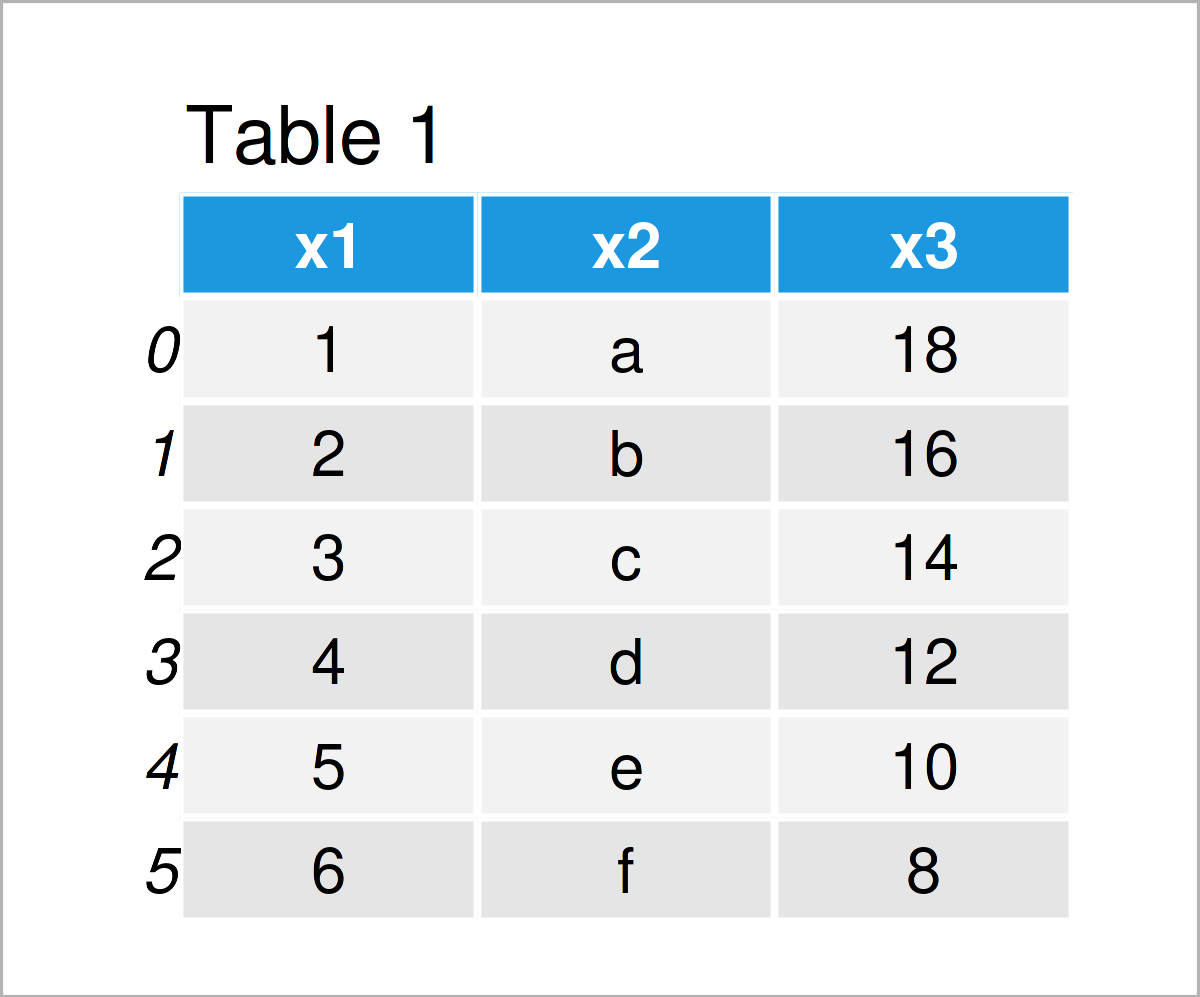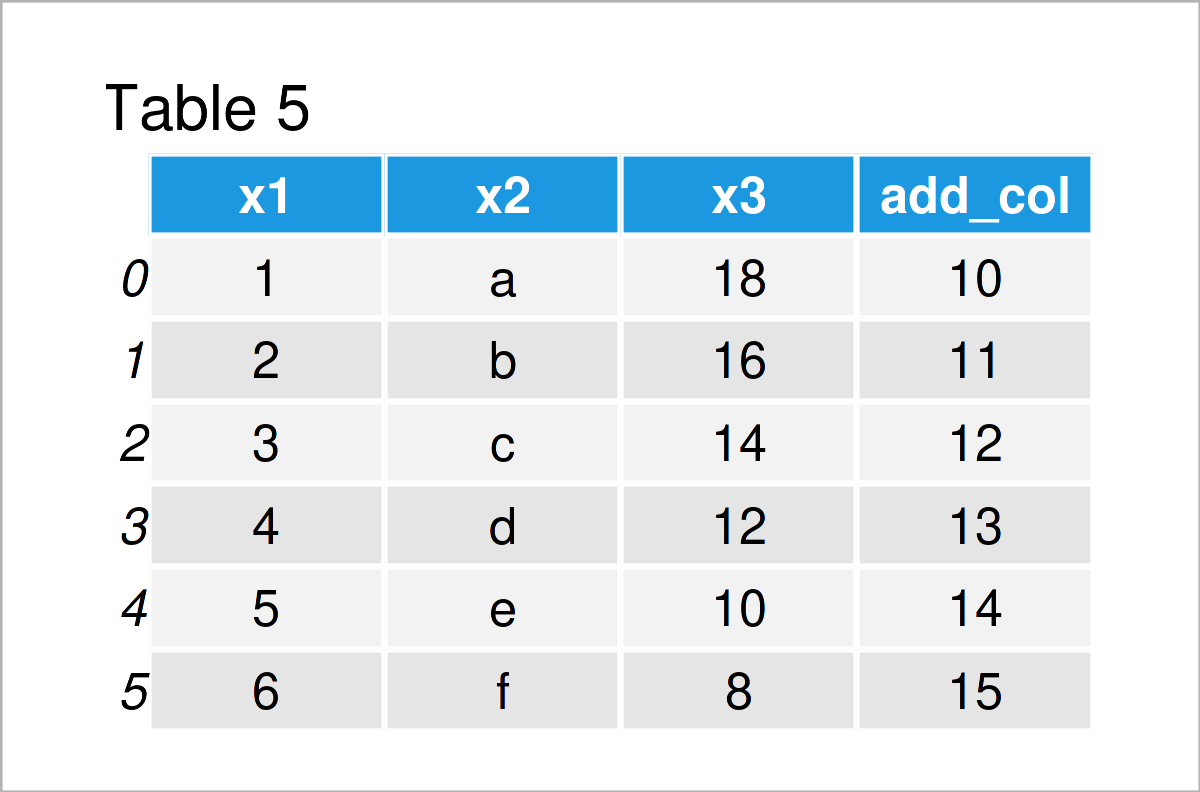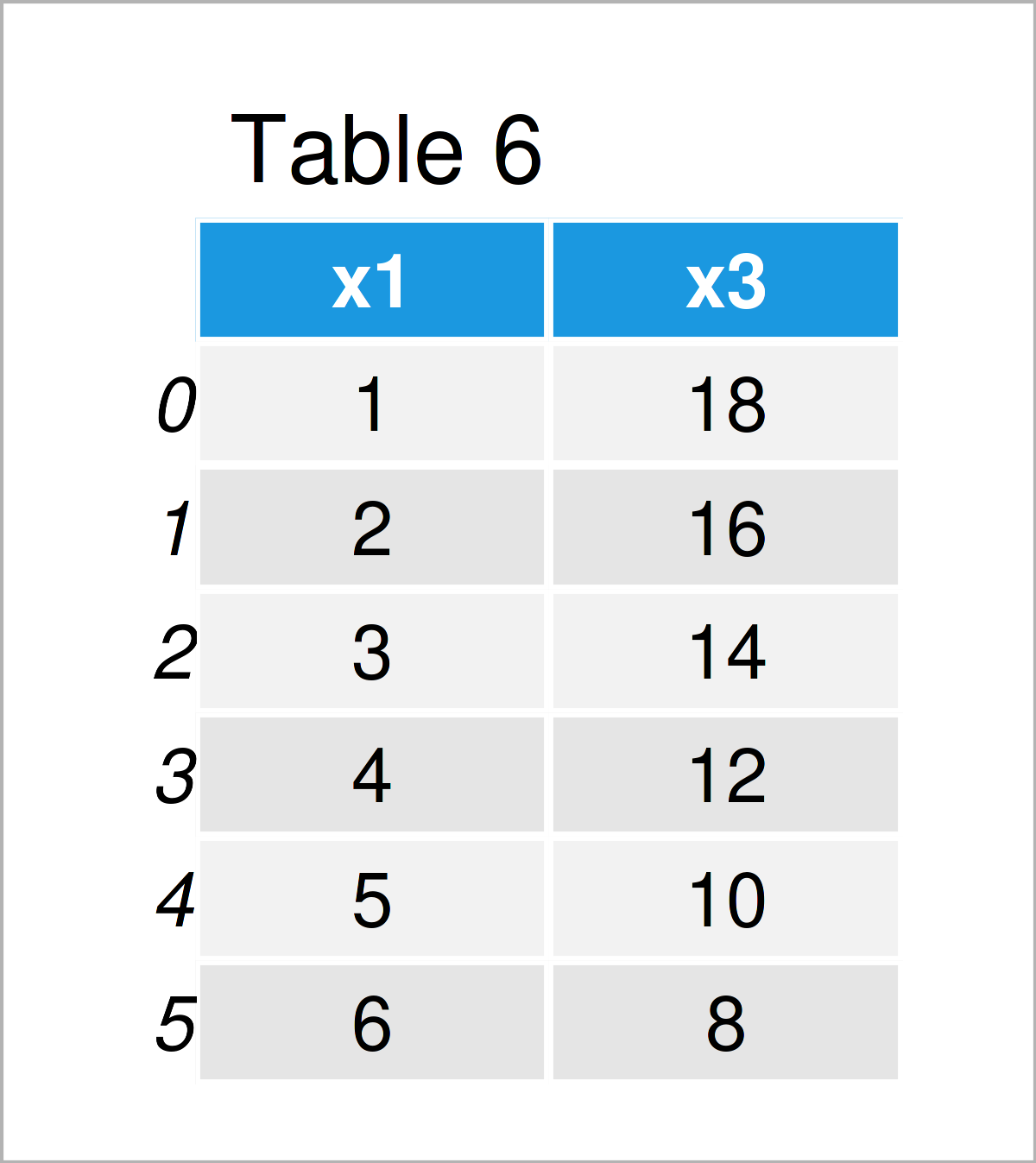# DataFrame Operations Using pandas in Python (5 Examples)

In this post you’ll learn how to change pandas DataFrames in the Python programming language.

The post will consist of five examples for the adjustment of a pandas DataFrame. To be more precise, the article will consist of the following topics:

It’s time to dive into the programming part:

## Exemplifying Data & Add-On Libraries

First, we need to import the pandas library:

`import pandas as pd                                    # Import pandas library in Python`

We’ll also need to construct some example data.

```data = pd.DataFrame({"x1":range(1, 7),                 # Create pandas DataFrame
"x2":["a", "b", "c", "d", "e", "f"],
"x3":range(18, 6, - 2)})
print(data)                                            # Print pandas DataFrame```Have a look at the previous table. It shows that our example DataFrame consists of six rows and three columns.

## Example 1: Replace Values in pandas DataFrame

The following Python code demonstrates how to substitute particular values in a pandas DataFrame.

For this task, we can use the replace function as shown below:

```data_new1 = data.copy()                                # Copy DataFrame
data_new1["x2"] = data_new1["x2"].replace("c", "new")  # Exchange values
print(data_new1)                                       # Print new DataFrame```After running the previous Python syntax the pandas DataFrame shown in Table 2 has been created. As you can see, we have exchanged the value “x” in the column x2 by the new character string “new”.

## Example 2: Append Row to pandas DataFrame

In Example 2, I’ll demonstrate how to add a new row to a pandas DataFrame.

For this task, we first have to create a list object that we can add as a new line to our data set:

```add_row = ["xxx", "yyy", "zzz"]                        # Create new row
# ['xxx', 'yyy', 'zzz']```

In the next step, we can add this list at the bottom of our example DataFrame:

```data_new2 = data.copy()                                # Copy DataFrame
print(data_new2)                                       # Print new DataFrame```After executing the previous Python programming code the data matrix shown in Table 3 has been created. As you can see, we have concatenated our list at the tail of our DataFrame.

## Example 3: Drop Rows from pandas DataFrame

This example shows how to remove a row from a pandas DataFrame based on a logical condition in Python programming.

More precisely, we are deleting all rows where the variable x3 is smaller or equal to the value 12:

```data_new3 = data[data.x3 <= 12]                        # Delete rows
print(data_new3)                                       # Print new DataFrame```As shown in Table 4, we have created a pandas DataFrame subset containing only some of the rows of our input DataFrame using the previous code.

## Example 4: Add Column to pandas DataFrame

This example explains how to join a new column to a pandas DataFrame.

```add_col = [10, 11, 12, 13, 14, 15]                     # Create new column
# [10, 11, 12, 13, 14, 15]```

Next, we can apply the assign function to add this list as a new variable to our example DataFrame:

```data_new4 = data.assign(add_col = add_col)             # Add new column to DataFrame
print(data_new4)                                       # Print new DataFrame```As shown in Table 5, we have created a new data set containing our example data and our list as an additional column.

## Example 5: Delete Column from pandas DataFrame

In Example 5, I’ll illustrate how to drop a certain column from a DataFrame object.

To achieve this, we can use the drop function as shown in the following Python code:

```data_new5 = data.drop("x2", axis = 1)                  # Remove column
print(data_new5)                                       # Print new DataFrame```In Table 6 it is shown that we have created a pandas DataFrame where we have excluded the variable x2 using the previous Python programming code.

## Video & Further Resources

Have a look at the following video on my YouTube channel in case you want to learn more on how to manipulate and adjust DataFrames using the pandas library. I demonstrate the Python programming syntax of this article in the video.

In addition, you might read the other articles on my homepage. I have released numerous articles already.

This tutorial has shown how to perform operations on pandas DataFrames in the Python programming language. In case you have any additional comments or questions, don’t hesitate to let me know in the comments.

Subscribe to the Statistics Globe Newsletter